Packing efficiency is defined as the percentage of total space filled by the constituent particles in a crystal.

Packing efficiency in hcp and ccp structures:

Let us consider the unit cell of ccp in which sphere ABCDEFGH are occupied at corner. And sphere a,b,c,d,e,f are placed at centre of face.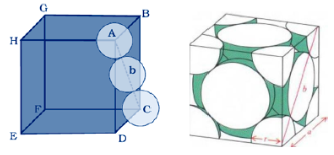Let the unit cell edge length be ‘a’ and face diagonal AC = b.

For calculation of packing efficiency consider the right angle triangle ABC. In triangle ABC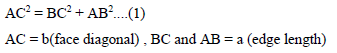Putting the value of AB, BC and AB in above eq..(1) we getIf r is the radius of the sphere, we find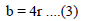By equating eq..(2) and (3) we get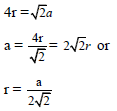Number of atom per unit cell in ccp = 4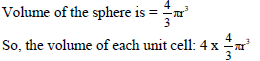Volume of cube = a3

Therefore,